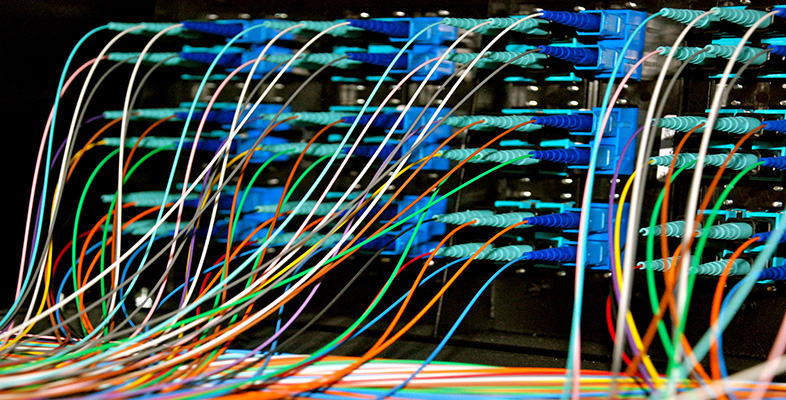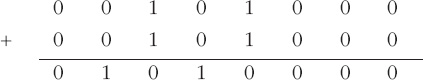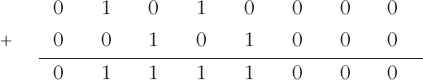Representing and manipulating data in computers

This free course is available to start right now. Review the full course description and key learning outcomes and create an account and enrol if you want a free statement of participation.

Free course

# 7.4 Multiplying 2's complement integers

Multiplication can be thought of as repeated addition. For instance, in denary arithmetic

7 × 5

can be thought of as

7 + 7 + 7 + 7 + 7

There is therefore no need for a new process for the multiplication of binary integers; multiplication can be transformed into repeated addition.

In multiplication the result is very often much larger than either of the two integers being multiplied, and so a multiple-length representation may be needed to hold the result of a multiplication. This is something that must be taken care of in any program that will be using multiplication.

In fact, there are some simple ways of reducing the number of steps in the multiplication process. I'll illustrate with a denary example.

To multiply 7 by 15, you could add 7 + 7 to get 14, then 14 + 7 to get 21, then 21 + 7 to get 28, and so on. You would carry out a total of 14 addition operations. Alternatively you could spot that 15 is 10 + 5 and that 7 × 10 is 70 which is simply 7 shifted one place to the left. So you could replace 9 of the additions with one shift to the left, and then do the final 5 additions. This would reduce a total of 14 operations to a total of 6 (one shift and five additions). The reduction in steps would be even more dramatic if the original sum was 7 × 105, because then a multiplication by 100 can be implemented by two shifts to the left (7 × 100 = 700) followed by 5 additions. This time 104 addition operations have been reduced to two shifts and five additions. Quite a saving!

Similarly, in binary arithmetic, a multiplication by two can be implemented by a shift one place to the left, a multiplication by four by two shifts to the left, and so on. Just as in denary arithmetic, the vacant space on the right is filled with a 0. So again a judicious mixture of shifts and additions can reduce the number of operations to be performed during a multiplication operation.

## Activity 26 (Exploratory)

1. Perform the binary multiplication 0010 1000 × 11 by adding 0010 1000 to itself the appropriate number of times.

2. Perform the binary multiplication 0010 1000 × 11 by first shifting 0010 1000 one place to the left and then adding 0010 1000.

3. Which would you say was simpler?

### Discussion

1. The first addition will give two (102) times 0010 1000:and the next addition will give three (112) times 0010 1000:So the result is 0111 1000.

2. Shifting 0010 1000 one place to the left (and filling up on the right with a 0) gives 0101 0000. Adding 0010 1000 to this gives (as before):I think the second operation was simpler. Do you agree?

T224_2